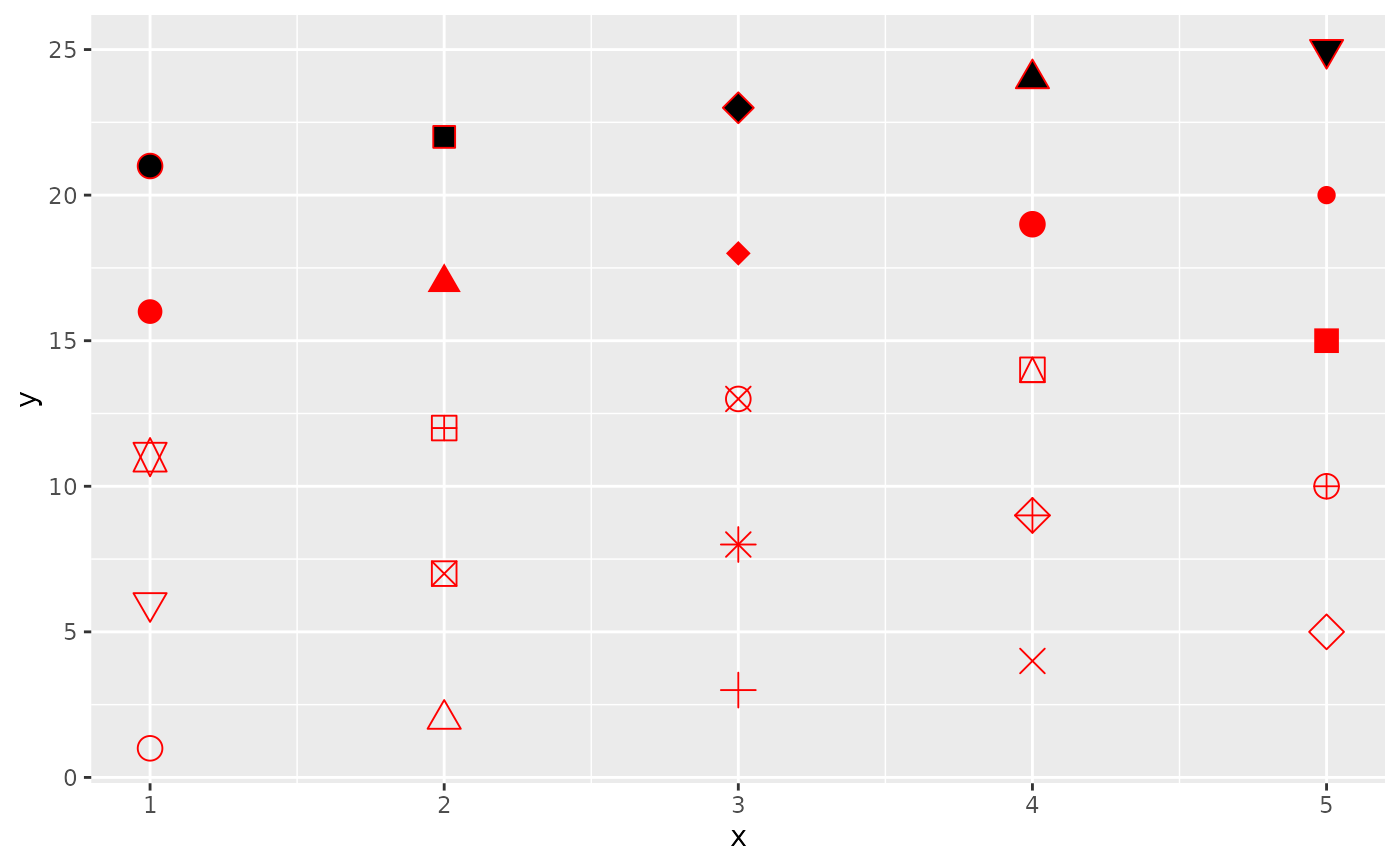The linetype, linewidth, size, and shape aesthetics modify the appearance of lines and/or points. They also apply to the outlines of polygons (linetype and linewidth) or to text (size).

## Linetype

The linetype aesthetic can be specified with either an integer (0-6), a name (0 = blank, 1 = solid, 2 = dashed, 3 = dotted, 4 = dotdash, 5 = longdash, 6 = twodash), a mapping to a discrete variable, or a string of an even number (up to eight) of hexadecimal digits which give the lengths in consecutive positions in the string. See examples for a hex string demonstration.

## Linewidth and stroke

The linewidth aesthetic sets the widths of lines, and can be specified with a numeric value (for historical reasons, these units are about 0.75 millimetres). Alternatively, they can also be set via mapping to a continuous variable. The stroke aesthetic serves the same role for points, but is distinct for discriminating points from lines in geoms such as geom_pointrange().

## Size

The size aesthetic control the size of points and text, and can be specified with a numerical value (in millimetres) or via a mapping to a continuous variable.

## Shape

The shape aesthetic controls the symbols of points, and can be specified with an integer (between 0 and 25), a single character (which uses that character as the plotting symbol), a . to draw the smallest rectangle that is visible (i.e., about one pixel), an NA to draw nothing, or a mapping to a discrete variable. Symbols and filled shapes are described in the examples below.

• geom_line() and geom_point() for geoms commonly used with these aesthetics.

• aes_group_order() for using linetype, size, or shape for grouping.

• Scales that can be used to modify these aesthetics: scale_linetype(), scale_linewidth(), scale_size(), and scale_shape().

• Run vignette("ggplot2-specs") to see an overview of other aesthetics that can be modified.

Other aesthetics documentation: aes_colour_fill_alpha, aes_group_order, aes_position, aes()

## Examples


df <- data.frame(x = 1:10 , y = 1:10)
p <- ggplot(df, aes(x, y))
p + geom_line(linetype = 2)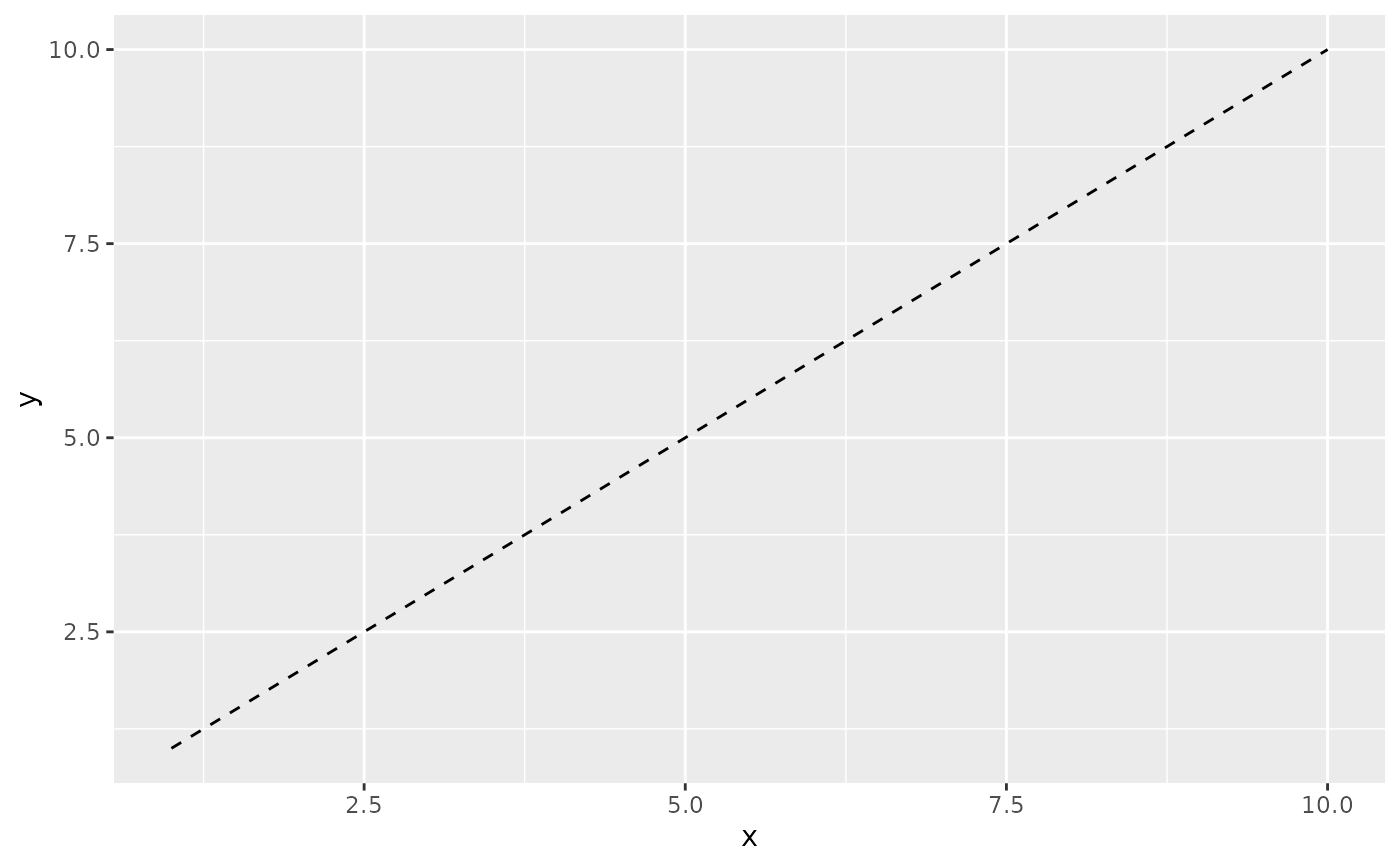p + geom_line(linetype = "dotdash")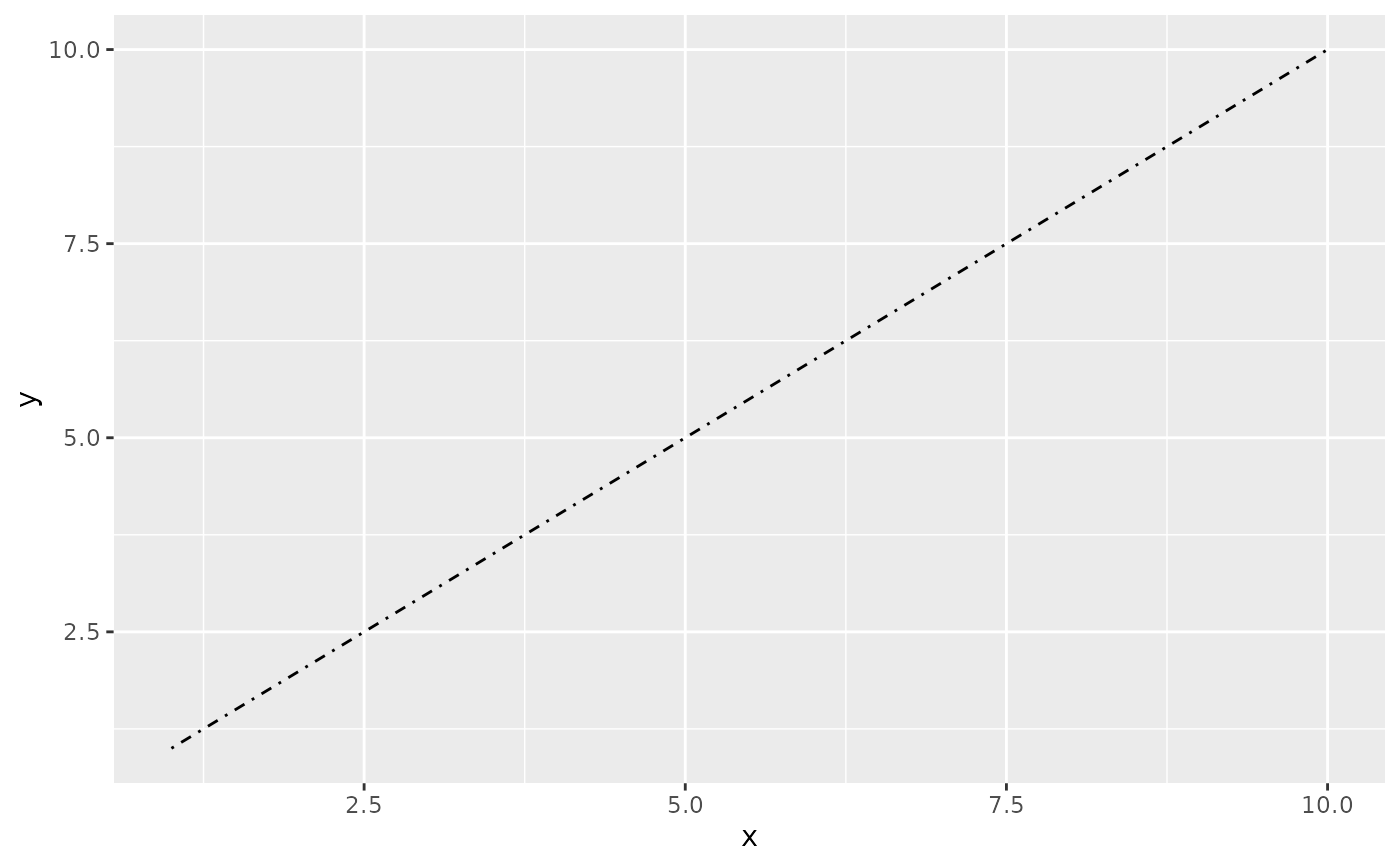# An example with hex strings; the string "33" specifies three units on followed
# by three off and "3313" specifies three units on followed by three off followed
# by one on and finally three off.
p + geom_line(linetype = "3313")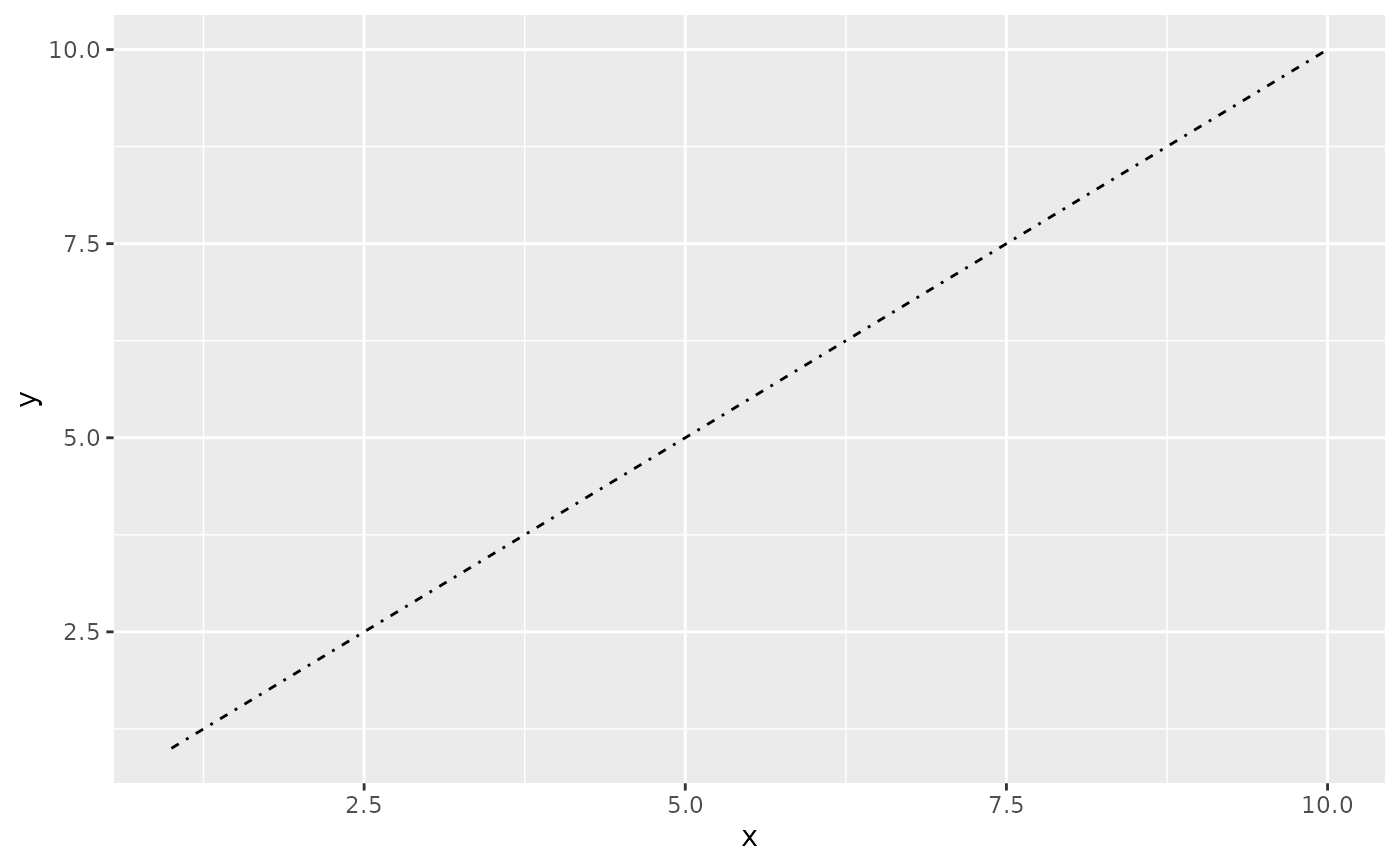# Mapping line type from a grouping variable
ggplot(economics_long, aes(date, value01)) +
geom_line(aes(linetype = variable))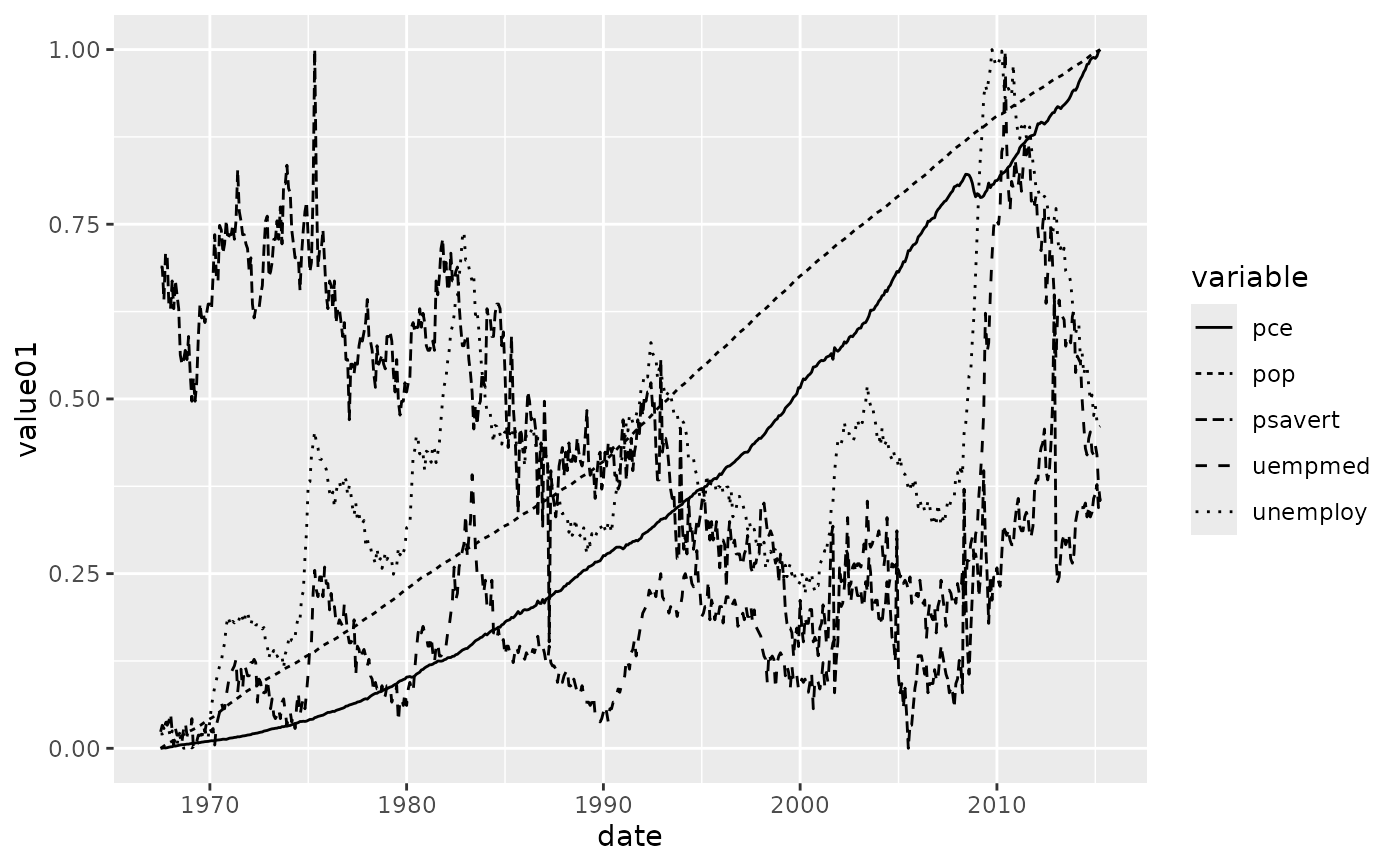# Linewidth examples
ggplot(economics, aes(date, unemploy)) +
geom_line(linewidth = 2, lineend = "round")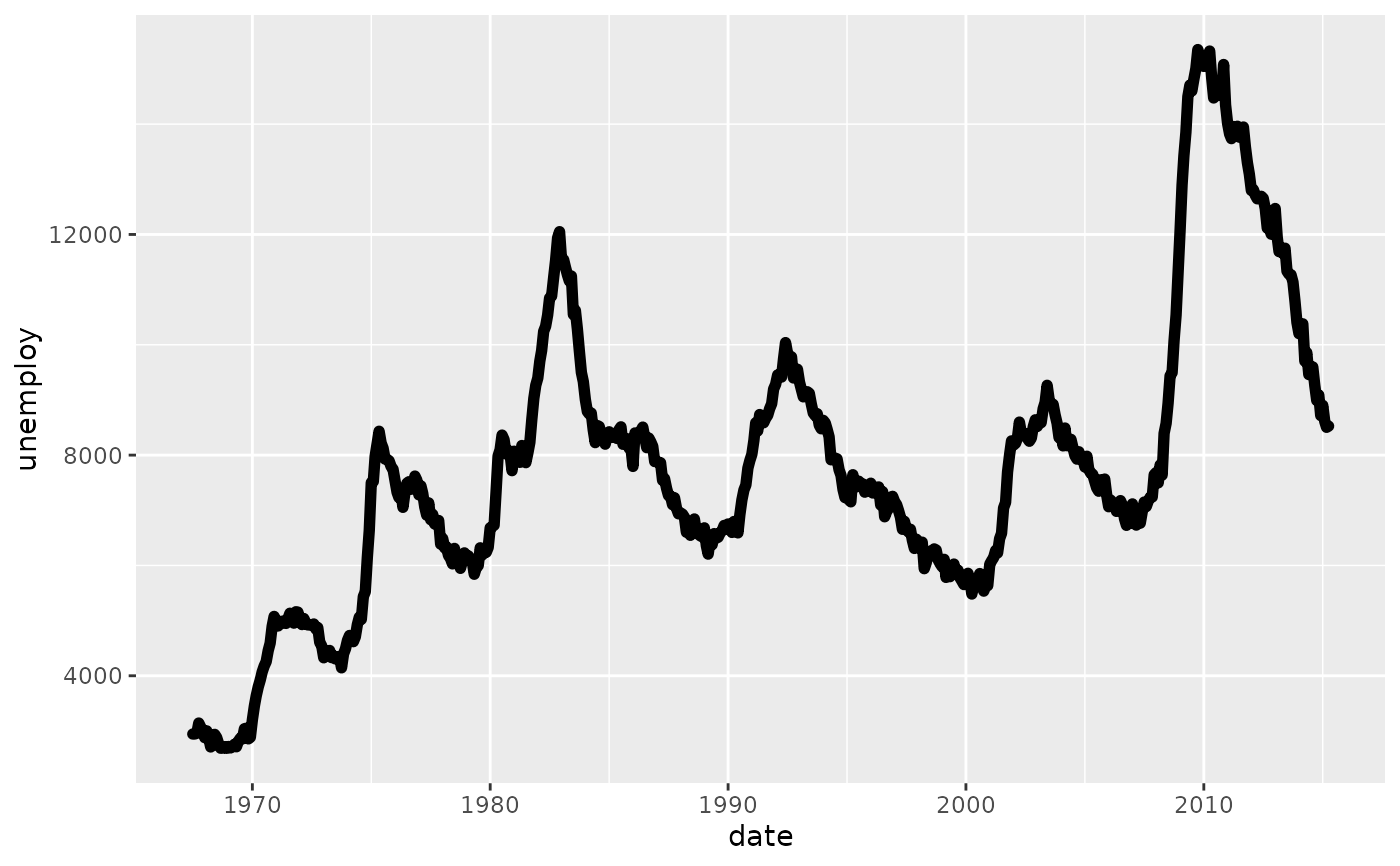ggplot(economics, aes(date, unemploy)) +
geom_line(aes(linewidth = uempmed), lineend = "round")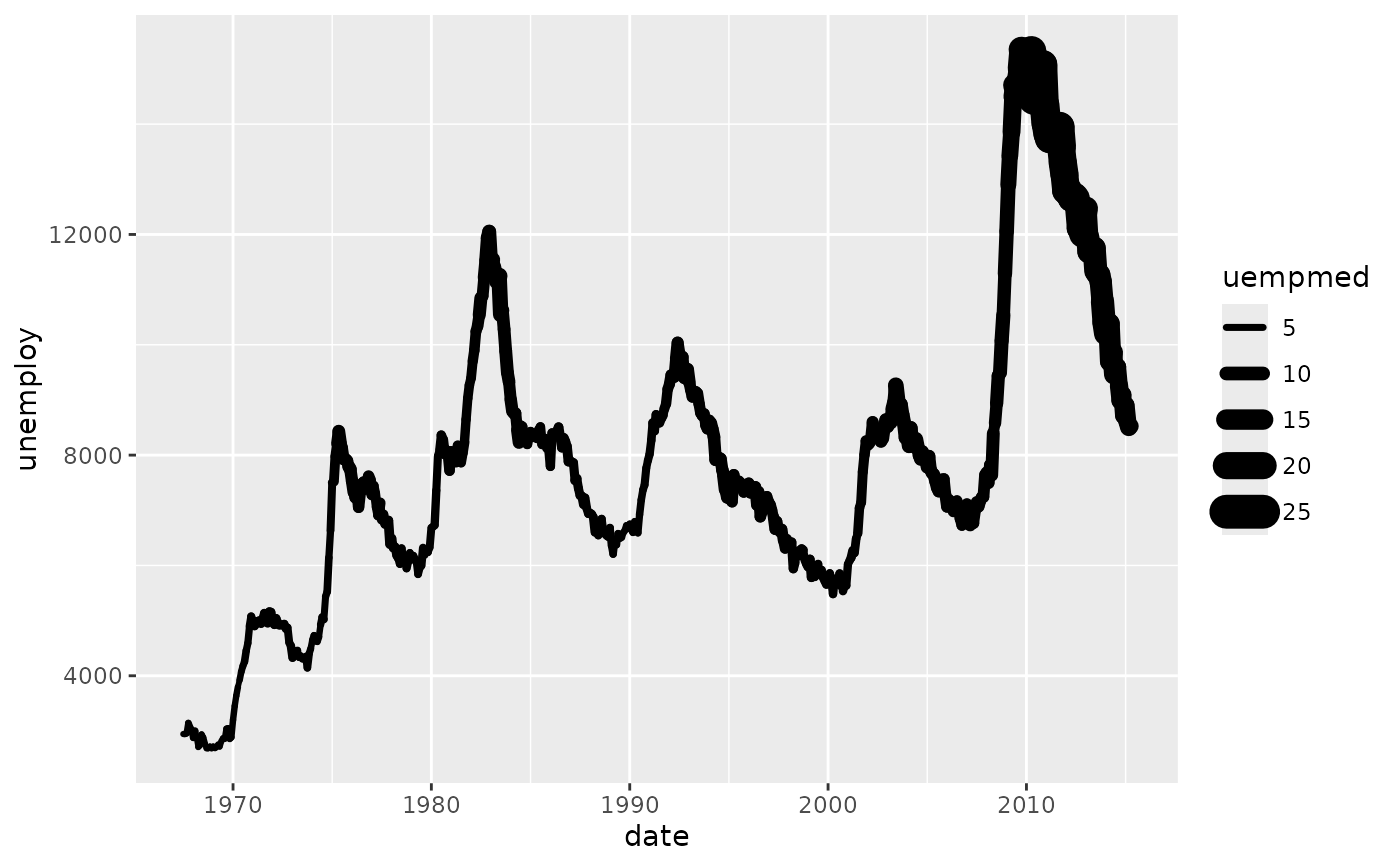# Size examples
p <- ggplot(mtcars, aes(wt, mpg))
p + geom_point(size = 4)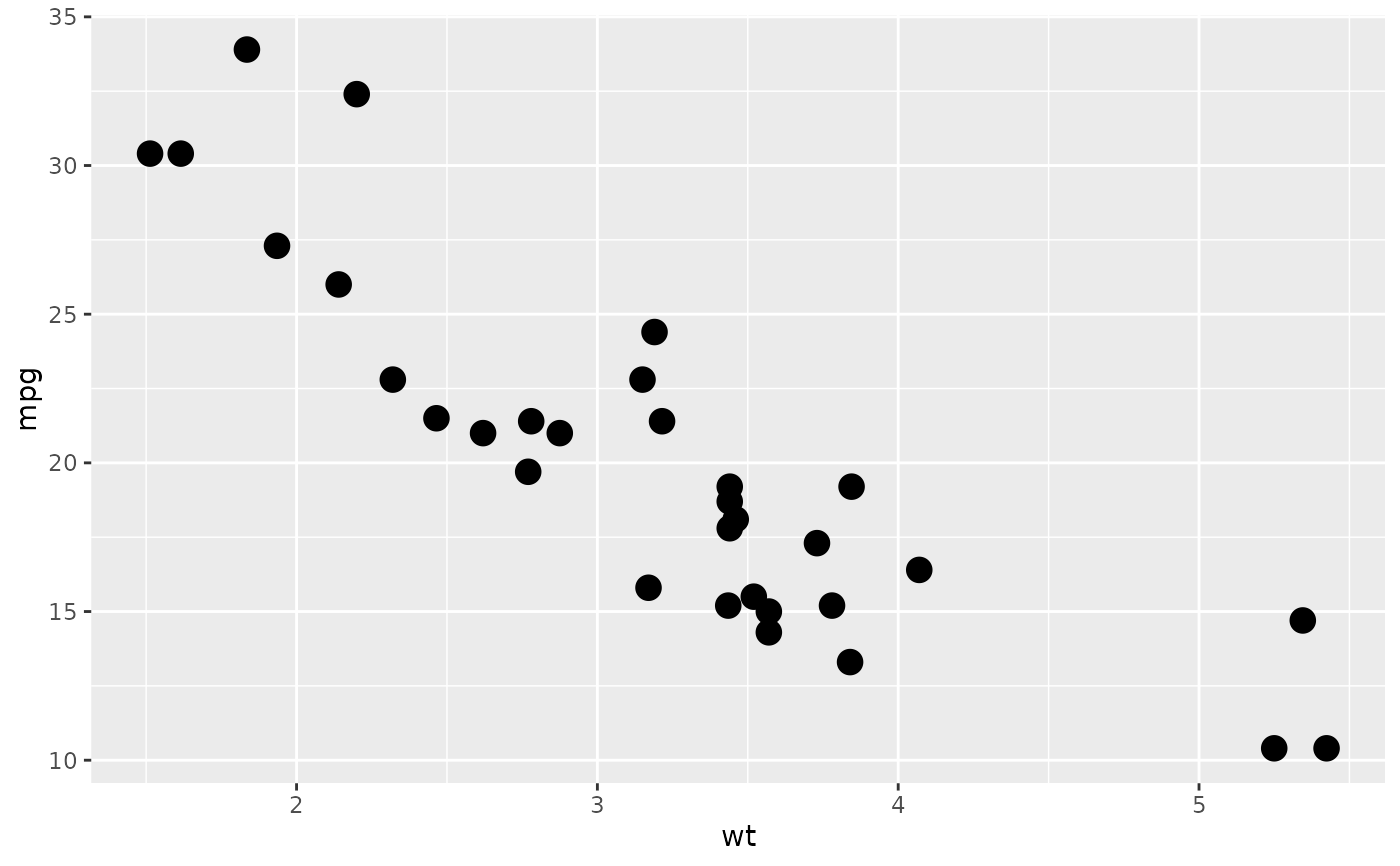p + geom_point(aes(size = qsec))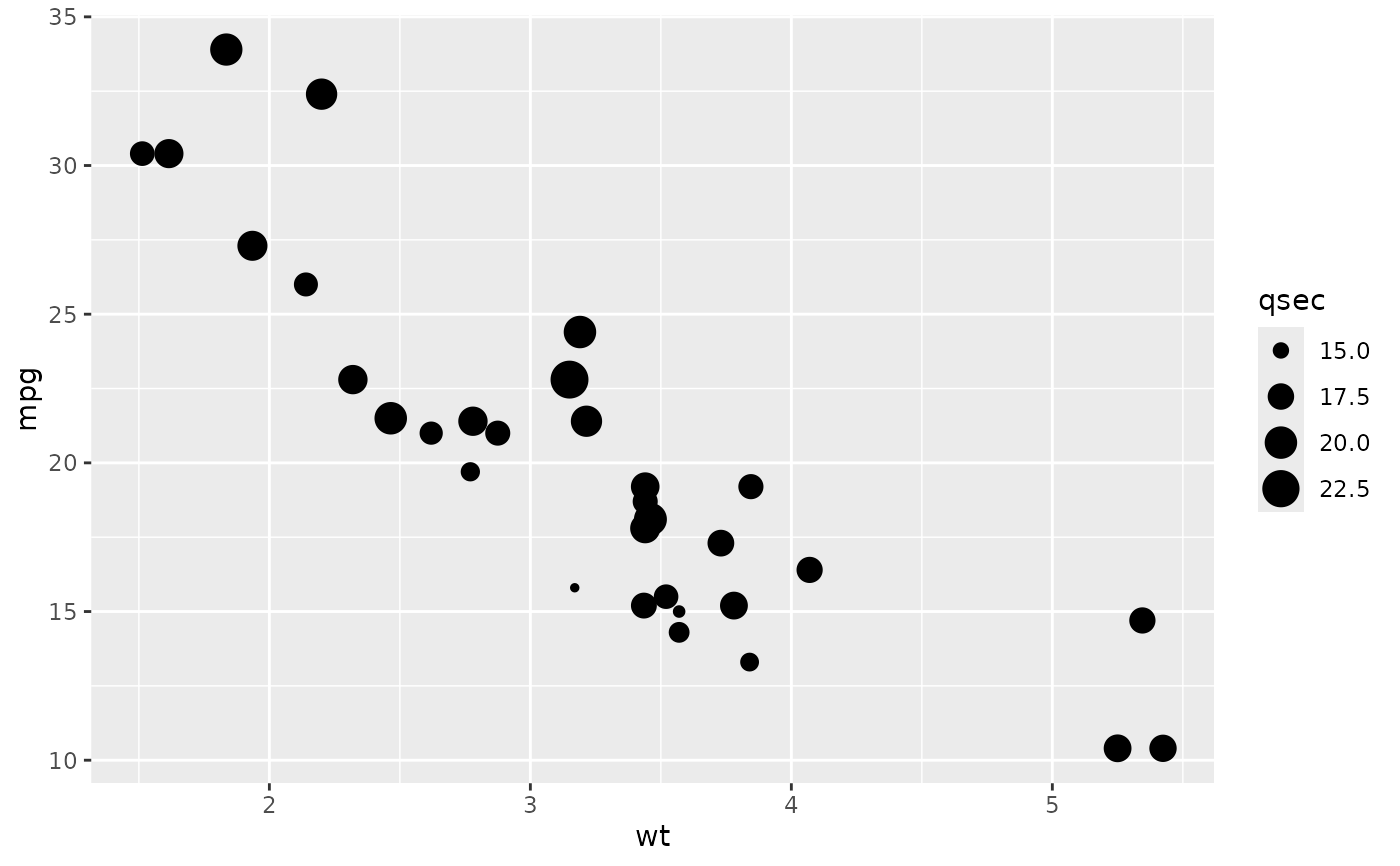p + geom_point(size = 2.5) +
geom_hline(yintercept = 25, size = 3.5)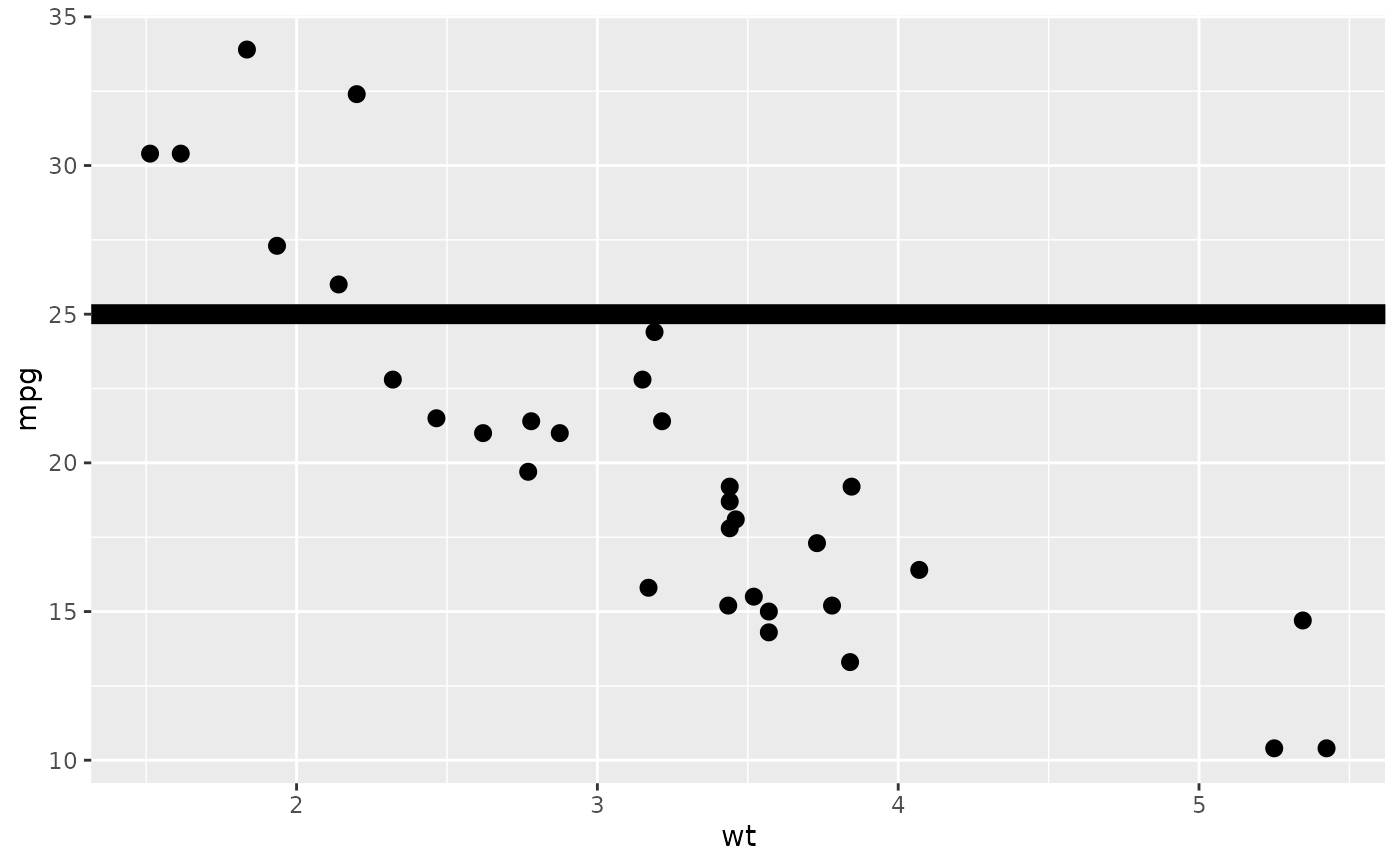# Shape examples
p + geom_point()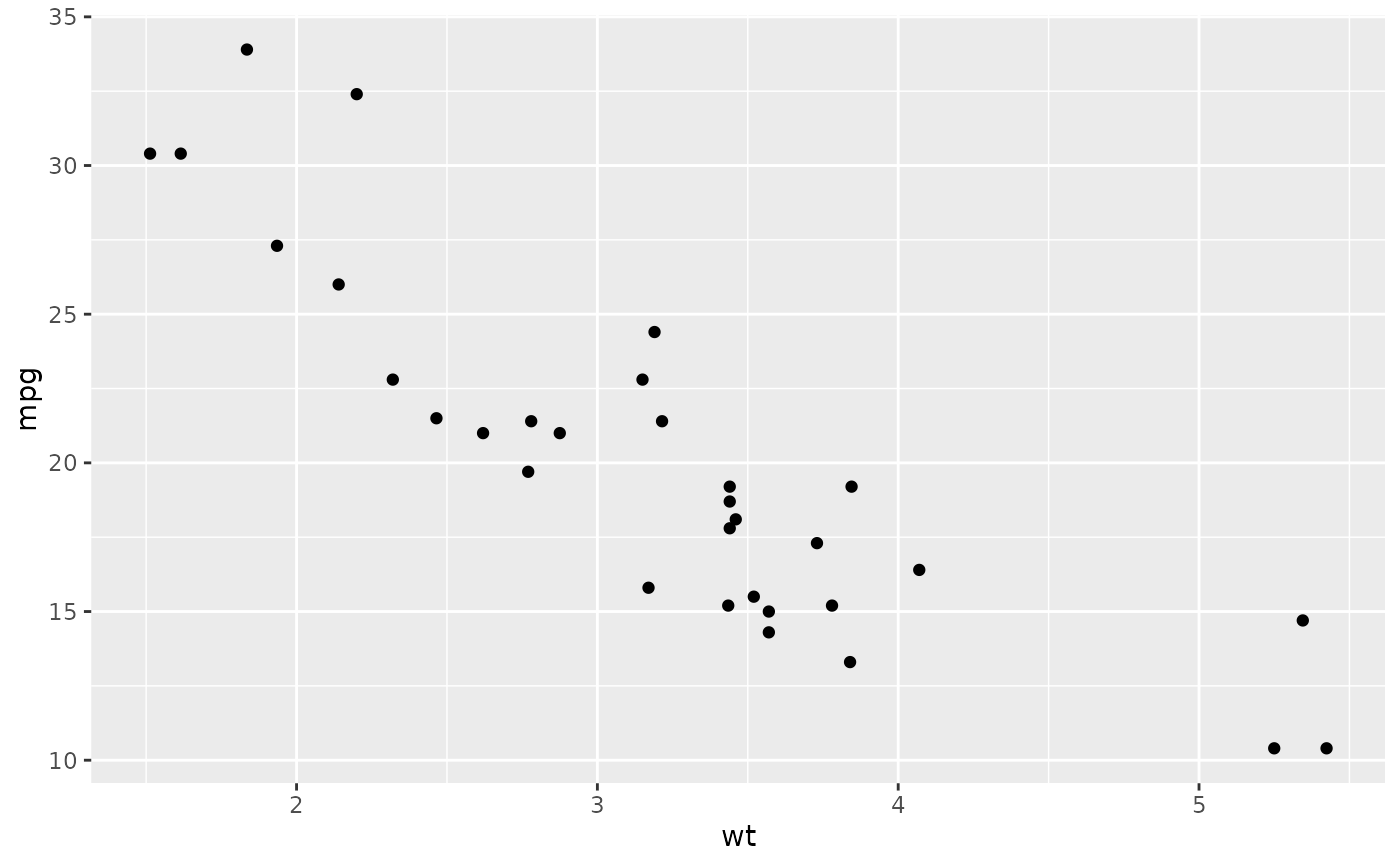p + geom_point(shape = 5)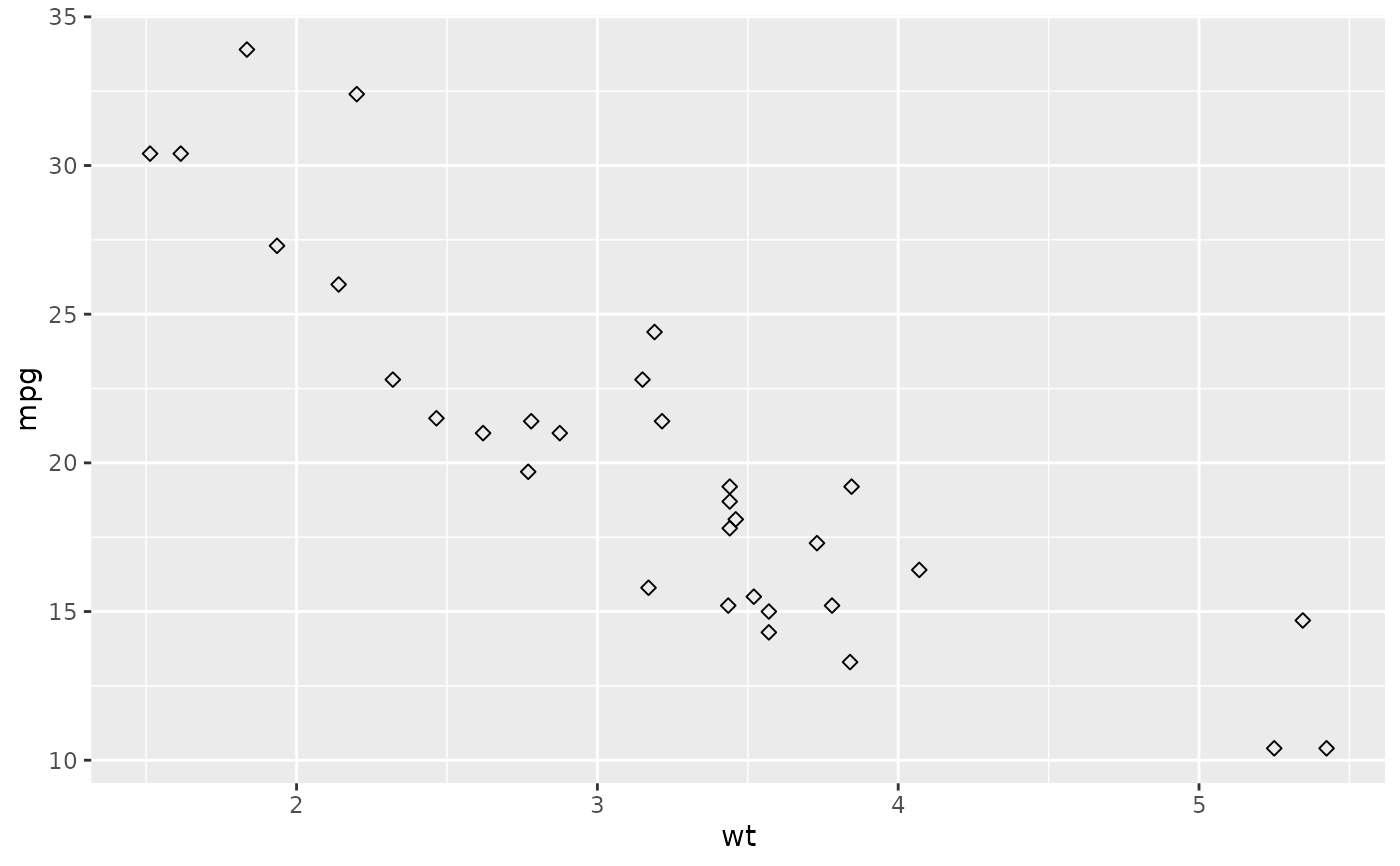p + geom_point(shape = "k", size = 3)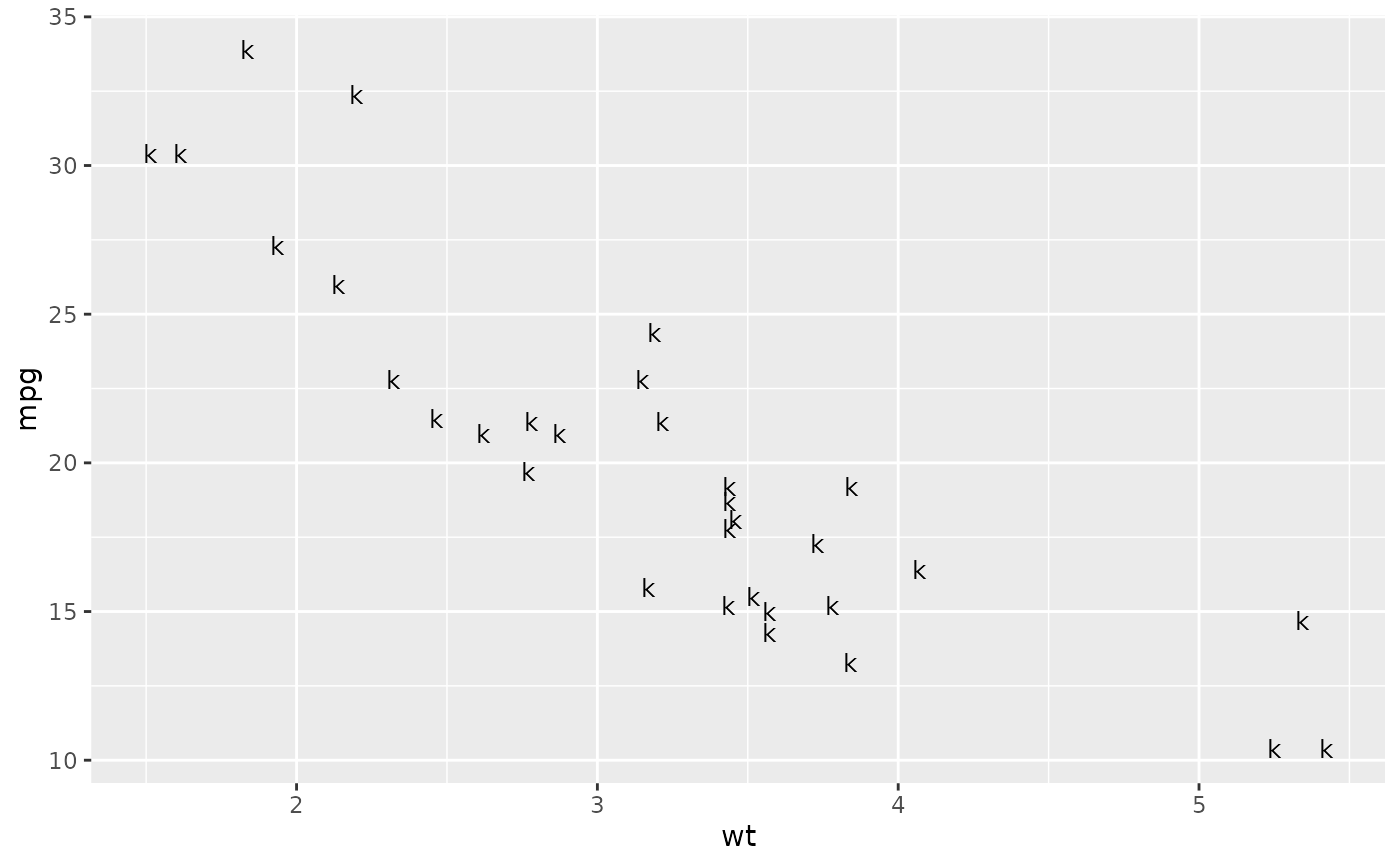p + geom_point(shape = ".")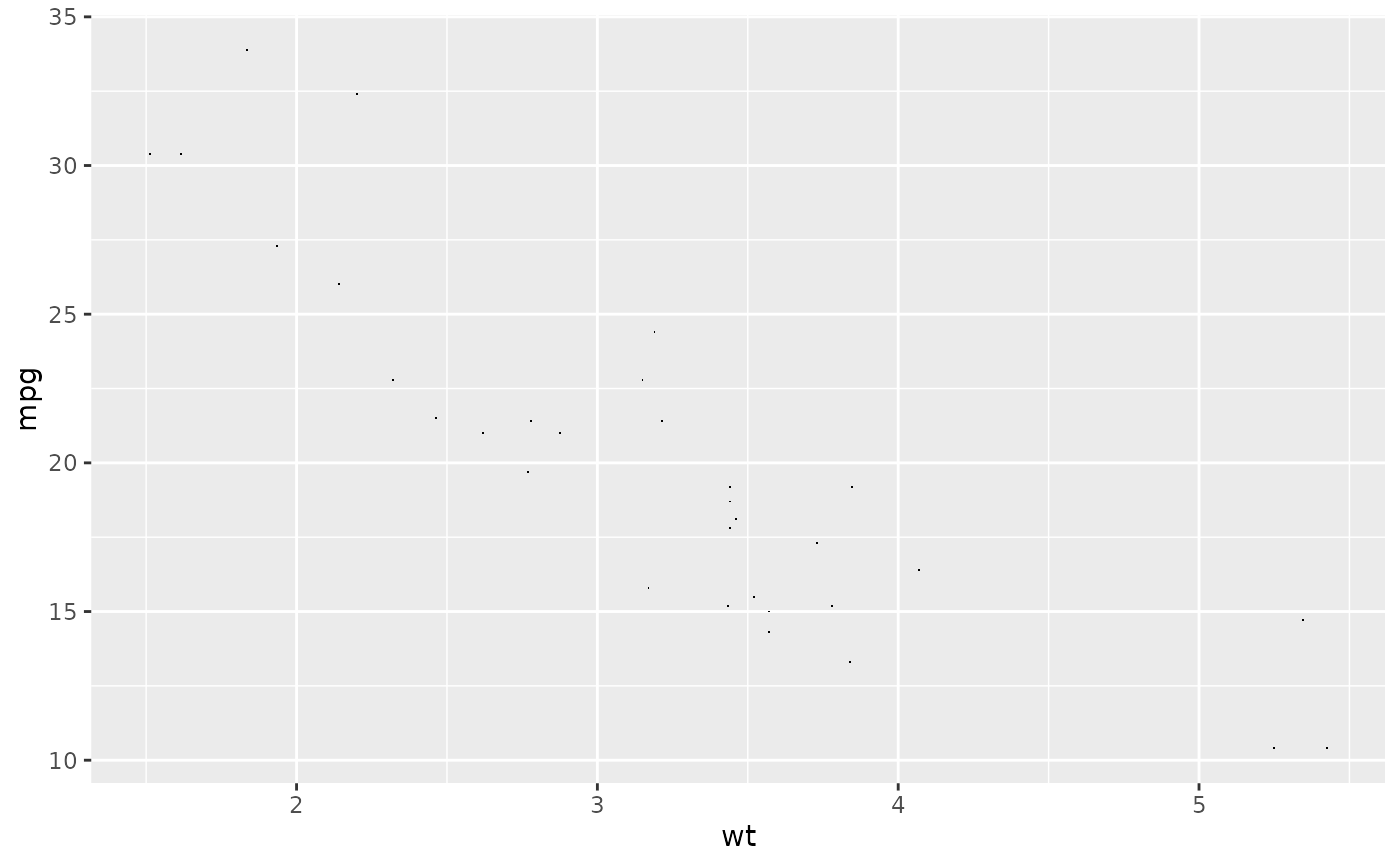p + geom_point(shape = NA)
#> Warning: Removed 32 rows containing missing values or values outside the scale
#> range (geom_point()).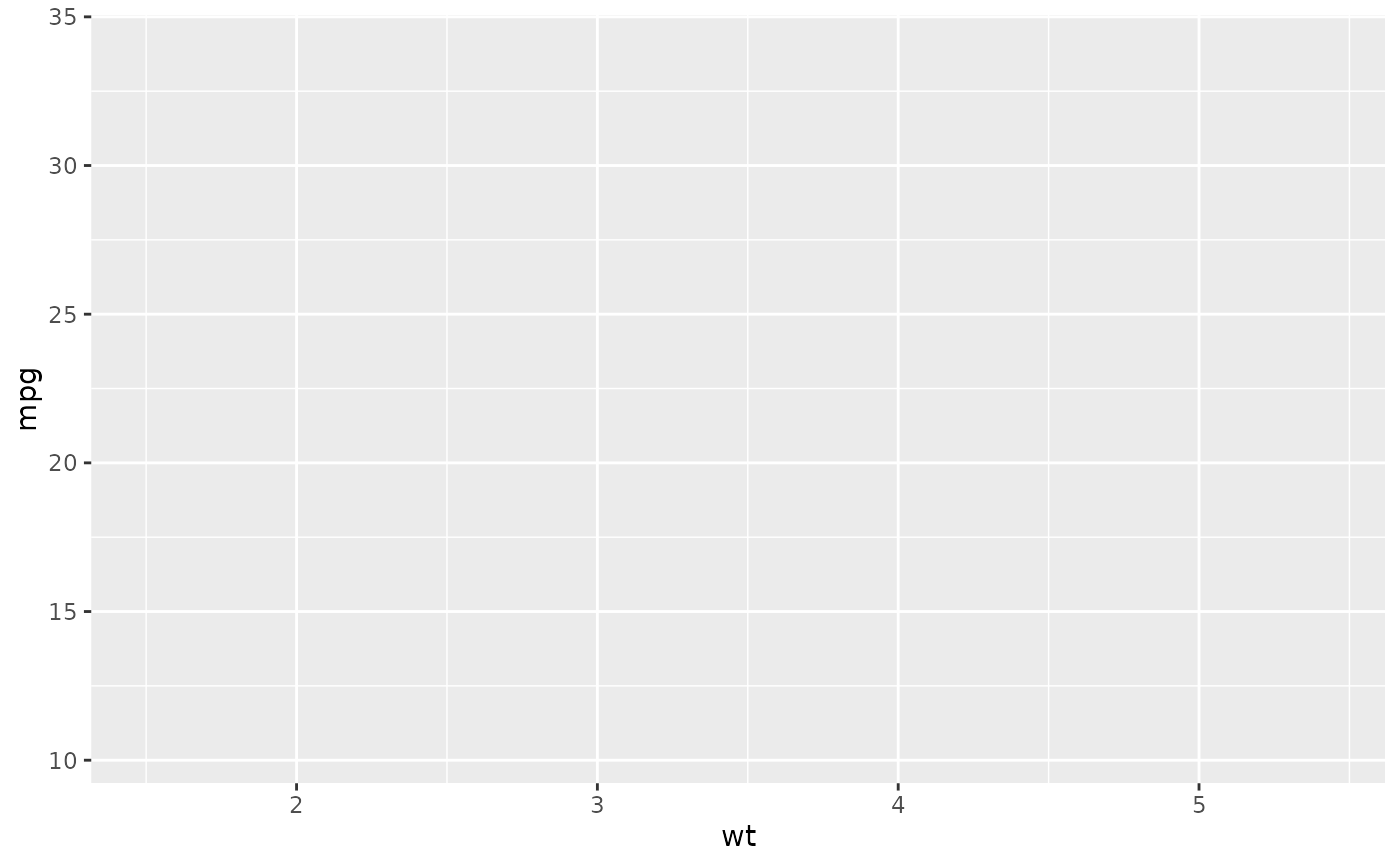p + geom_point(aes(shape = factor(cyl)))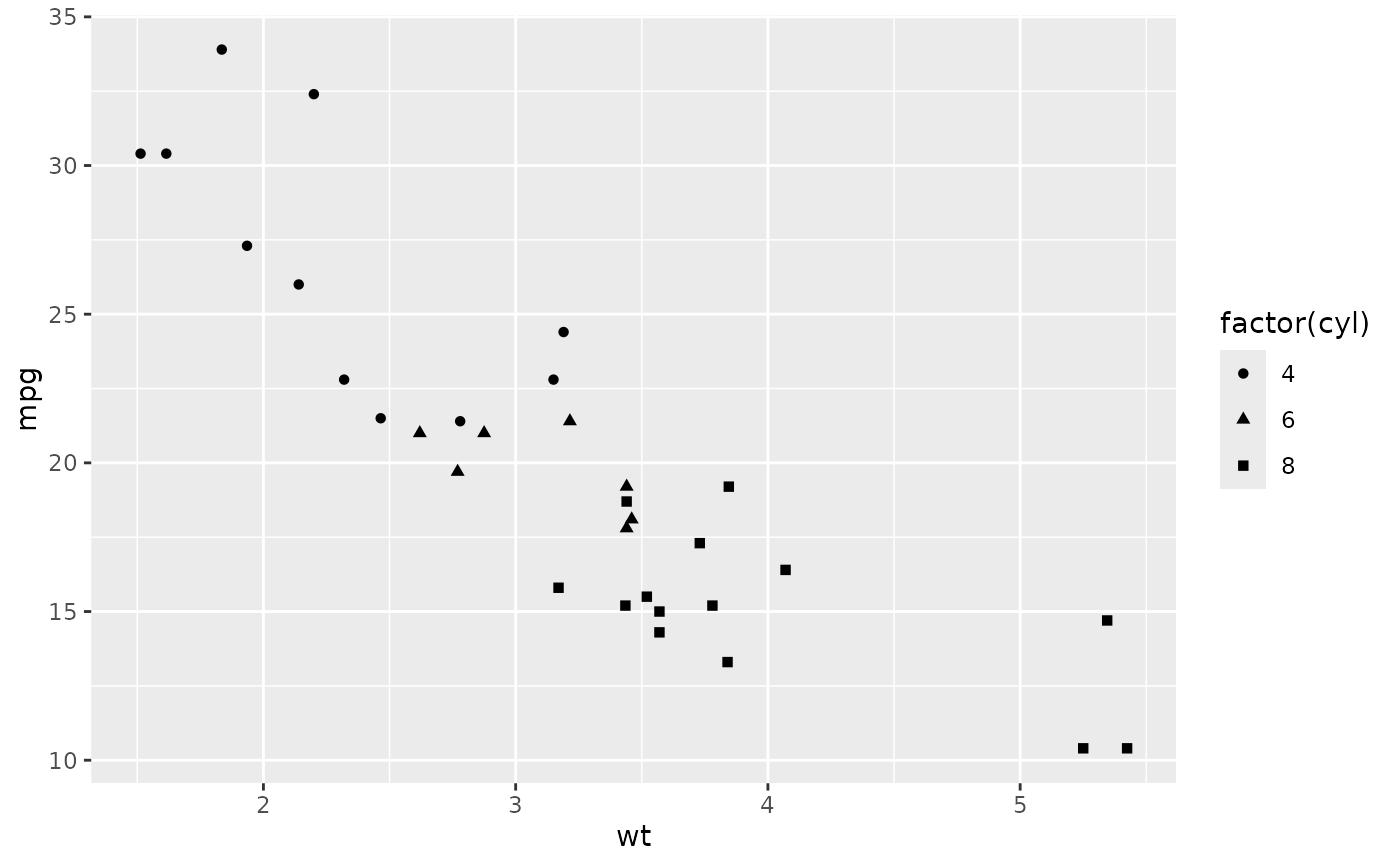# A look at all 25 symbols
df2 <- data.frame(x = 1:5 , y = 1:25, z = 1:25)
p <- ggplot(df2, aes(x, y))
p + geom_point(aes(shape = z), size = 4) +
scale_shape_identity()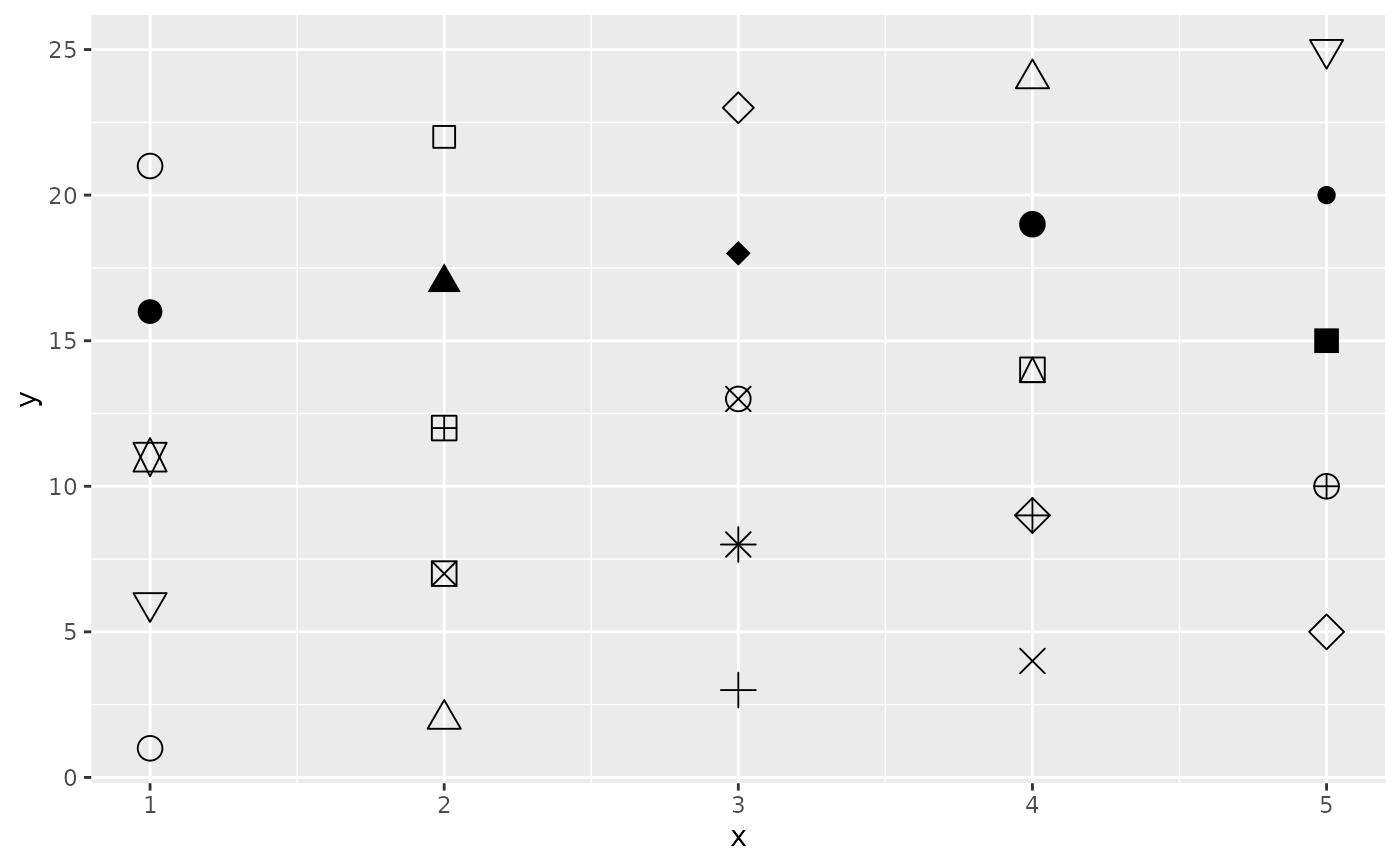# While all symbols have a foreground colour, symbols 19-25 also take a
# background colour (fill)
p + geom_point(aes(shape = z), size = 4, colour = "Red") +
scale_shape_identity()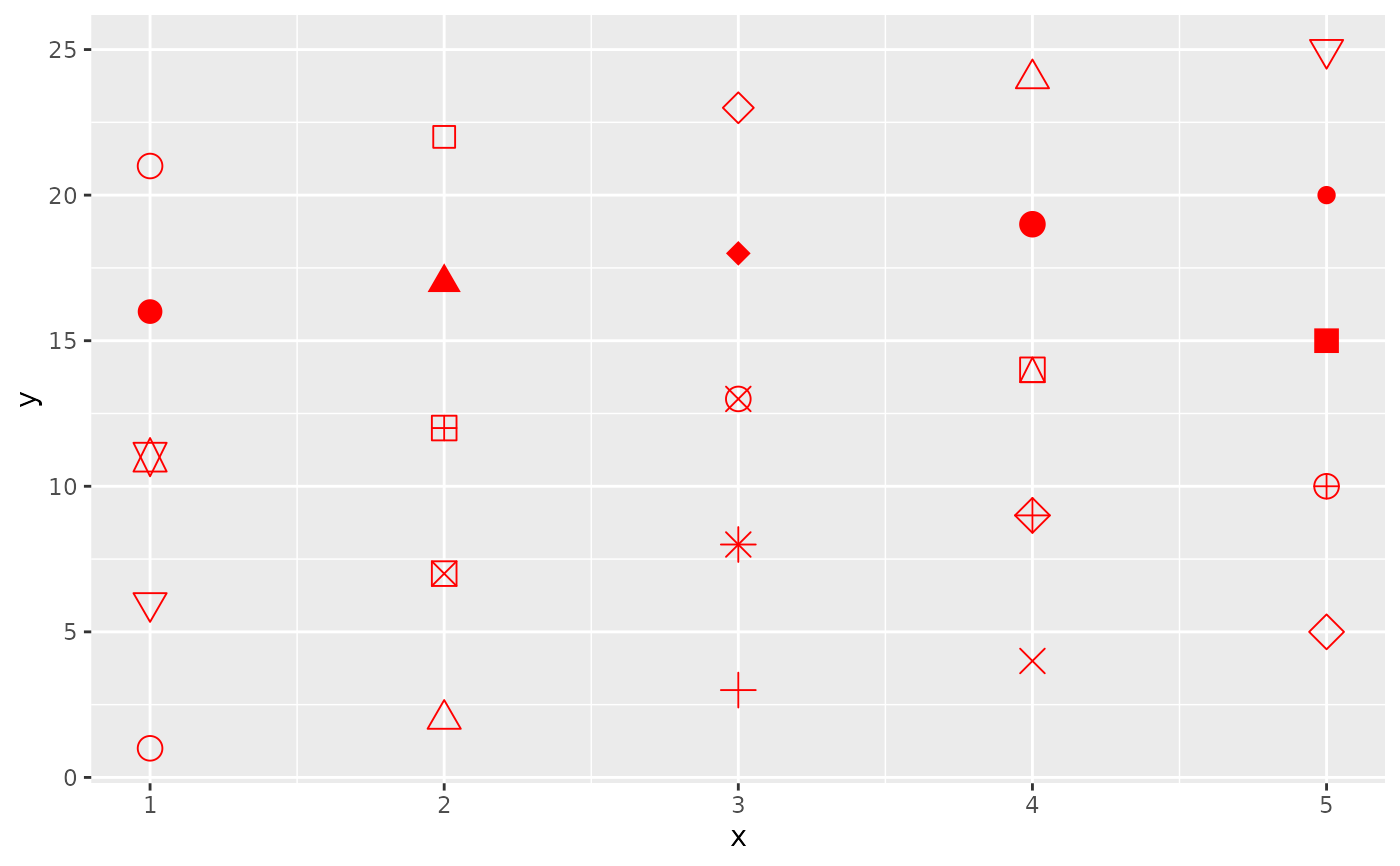p + geom_point(aes(shape = z), size = 4, colour = "Red", fill = "Black") +
scale_shape_identity()